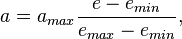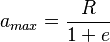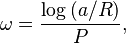# Galaxy Structure

A galaxy is made up of stars, gas, dust, and other material gravitationally bound together. It is believed that most if not all galaxies have a supermassive black holes at their centers. In Universe Sandbox, every Galaxy object is made up of a number of Galaxy Nebula objects orbiting a single Black Hole object at the center. Each Galaxy Nebula object represents a collection of stars, gas, and dust orbiting together.

Some galaxies have complex structures, while others appear as amorphous blobs of material with no structure at all. Galaxies are classified according to their observed structure. The nebulae in Galaxy objects in Universe Sandbox are placed into orbits around the black hole that reproduce the expected structure based on the Galaxy's type.

## Models

A Galaxy in Universe Sandbox is simulated as a number of non-attracting objects, the nebulae, orbiting a single attracting object, the black hole. This is a rough approximation of the way gravity works in a real galaxy (see Limitations for details), where most of the mass is located in the stars and nebulae, not in the central black hole. To maintain the correct orbital periods for the simulated nebulae, the N-body simulation uses the total mass of the galaxy in place of the black hole's actual mass to calculate the motion of the nebulae.

### Irregular Galaxies

The positions of the Galaxy Nebula objects in an irregular galaxy are randomly chosen such that their x, y, and z-components all lie between -R and R, where R is the radius of the Galaxy object. The velocity of each nebula is then calculated such that the nebula is on a circular orbit around the central Black Hole object.

### Elliptical Galaxies

In Universe Sandbox, an elliptical galaxies is simulated as a spheroids, or a sphere in which one axis (the minor axis) may be shorter than the longest axis (or major axis) of the galaxy. The major axis is set equal to the radius of the galaxy, R, and the minor axis is set so that the ratio of the minor to major axes is equal to the Elliptical Ratio of the galaxy. A sphere will have an Elliptical Ratio of 1, and the major and minor axes will both equal the galaxy's radius.

The orbital elements of the Galaxy Nebula objects in an elliptical galaxy are randomly chosen from within the following ranges:

The Eccentricity of each nebula is set to 0.01, and the Argument of Pericenter is set to 0.

The Semi-Major Axis is then randomly chosen from within a distribution with a maximum possible value of$R_{max} = \frac{AB}{A^2 \sin^2 i + B^2 \cos^2 i},$

where A and B are the major and minor axes of the galaxy, respectively, and i is the inclination of the nebula. This ensures that the object orbits within the elliptical shape of the galaxy. Unlike the other orbital elements, the Semi-Major Axis is not selected from a uniform distribution in this range, but rather from a Sérsic distribution with an index of 1.

### Spiral Galaxies

In Universe Sandbox, a spiral galaxy is simulated in two parts: a central bulge, which resembles a spherical elliptical galaxy, and a larger, flat, disk, extending out to the radius of the galaxy. The size of the bulge relative to the disk is determined by the type of spiral. The orbital elements of the nebulae in the bulge are randomly selected in the method described above for elliptical galaxies, except that the eccentricities of the particles are set to 0 rather than 0.01, and the index of the Sérsic distribution is 4 rather than 1.

For nebulae in the disk of the galaxy, the Eccentricity is randomly chosen from a distribution between emin=0.2 and emax=0.5. The Semi-Major Axis is then calculated with$a = a_{max}\frac{e - e_{min}}{e_{max}-e_{min}},$

where$a_{max}=\frac{R}{1+e}$

is the maximum possible Semi-Major Axis for a particle with the given Eccentricity. This ensures that the eccentricities of the nebulae will increase with increasing distance from the bulge.

The Argument of Pericenter for a nebula in the bulge is calculated using$\omega = \frac{\log{(a/R)}}{P},$

where P is the Pitch Angle of the galaxy. This rotation of the orbits based on the semi-major axis creates the spiral arm structure. To create more than one spiral arm, the Argument of Pericenter of a fraction of the nebulae are rotated by increments of 360/n degrees, where n is the number of spiral arms in the galaxy. To simulate the imperfections in the arms of real spiral galaxies, an additional number of degrees, ωnoise, is added to the Argument of Pericenter for each nebula, where ωnoise is randomly selected within a maximum equal to 180 degrees times the Spiral Arm Noise property of the galaxy.

## Limitations

### N-Body Simulation

In real galaxies, only a small fraction of the mass of the galaxy exists in the central black hole; most is distributed among the stars and other material. This is in contrast to the Solar System and other planetary systems, where the majority of the mass is located in the central star or stars. To capture the complex gravitational interactions between the stars in a galaxy, Universe Sandbox would need to make all of the nebulae objects attracting. But the appearance of a Galaxy object depends on having a large number of nebulae objects (hundreds to thousands), and including this many attracting objects in a simulation would have a drastically negative effect on performance. Instead, Universe Sandbox approximates the structure of galaxies using the model described above, with non-attracting nebulae objects. This approximation allows Universe Sandbox to create galaxies with structures like spiral arms, but not features that depend on the gravitational attraction between stars, like the bars in barred spiral galaxies.

### Dark Matter

The motion of stars in the outer regions of spiral galaxies indicates the presence of more mass than is observed, based on the measured light from the galaxies. This "missing mass" has been termed dark matter, for its apparent lack of interaction with light. Several theories have been proposed to explain the nature of dark matter, including the existence of some type of particle that interacts with other matter via gravity but does not interact with light.

Previous versions of Universe Sandbox (pre-Update 23) included Dark Matter objects to simulate this effect. However, when the improved Galaxy model was released in Update 23, dark matter simulation was removed, and the developers explained their reasoning in a blog post: the simplified model of nebulae orbits in a galaxy cannot reproduce the observed motion in real galaxies that prompted the theory of dark matter, so Dark Matter objects cannot simulate the main effect of dark matter in galaxies.